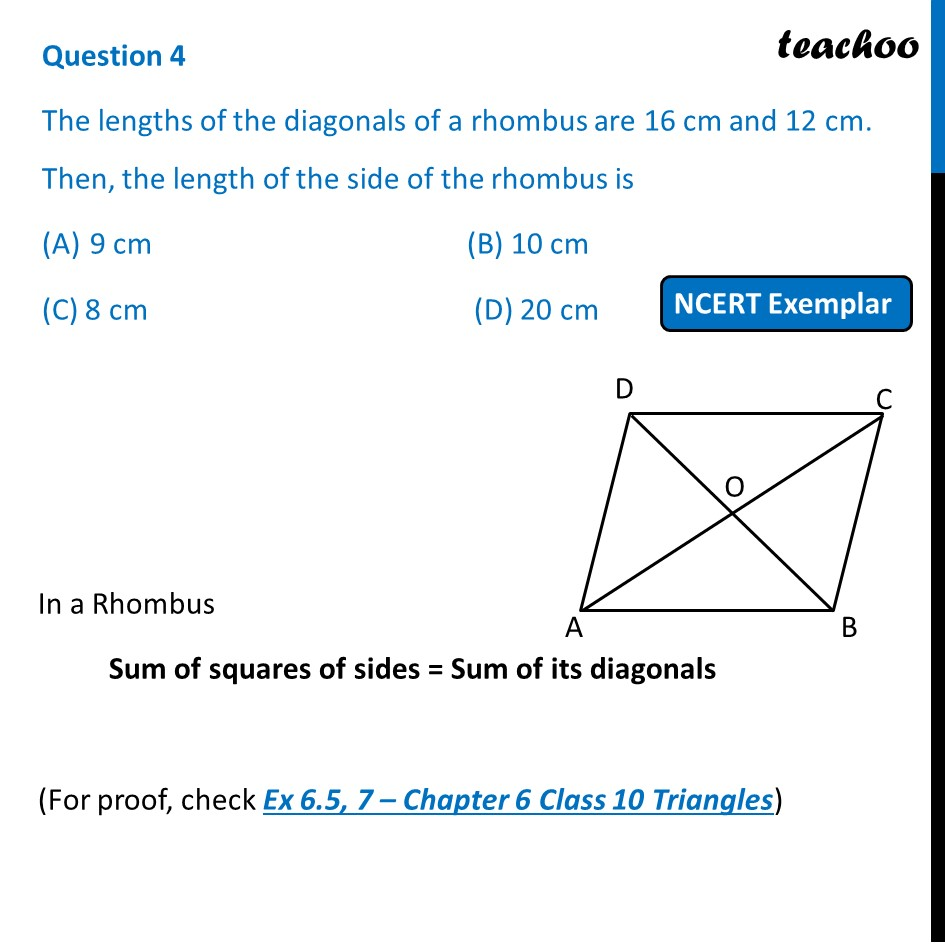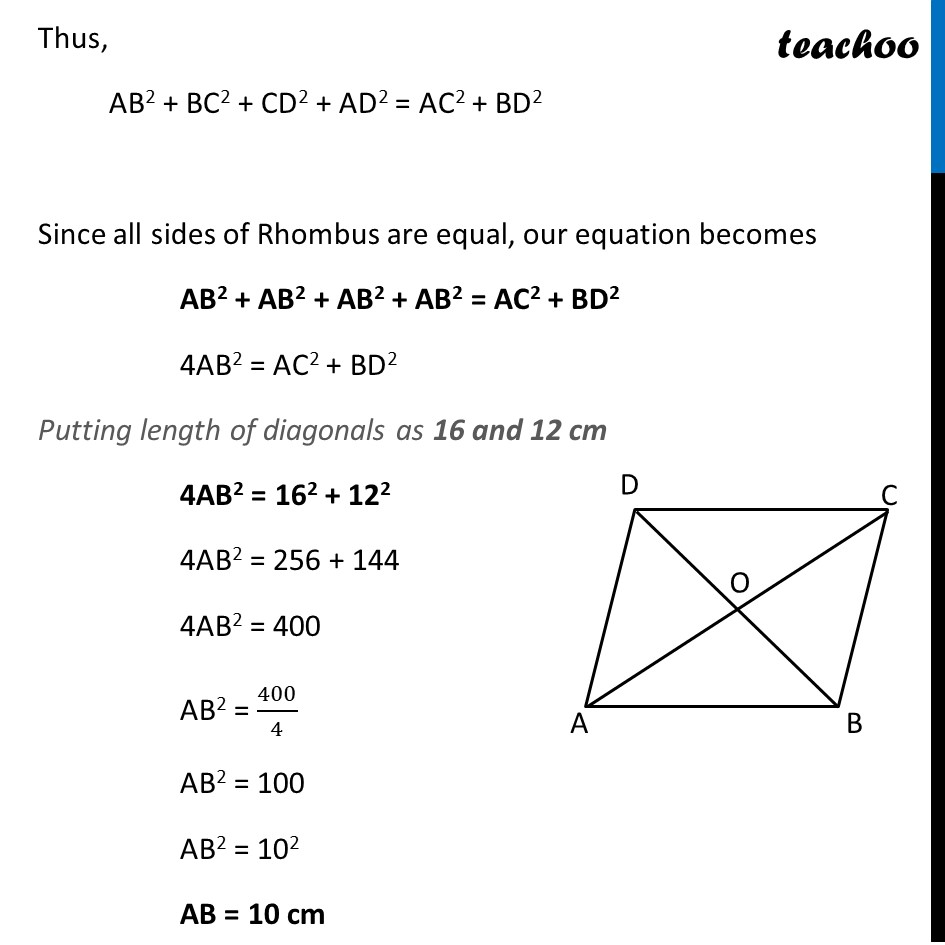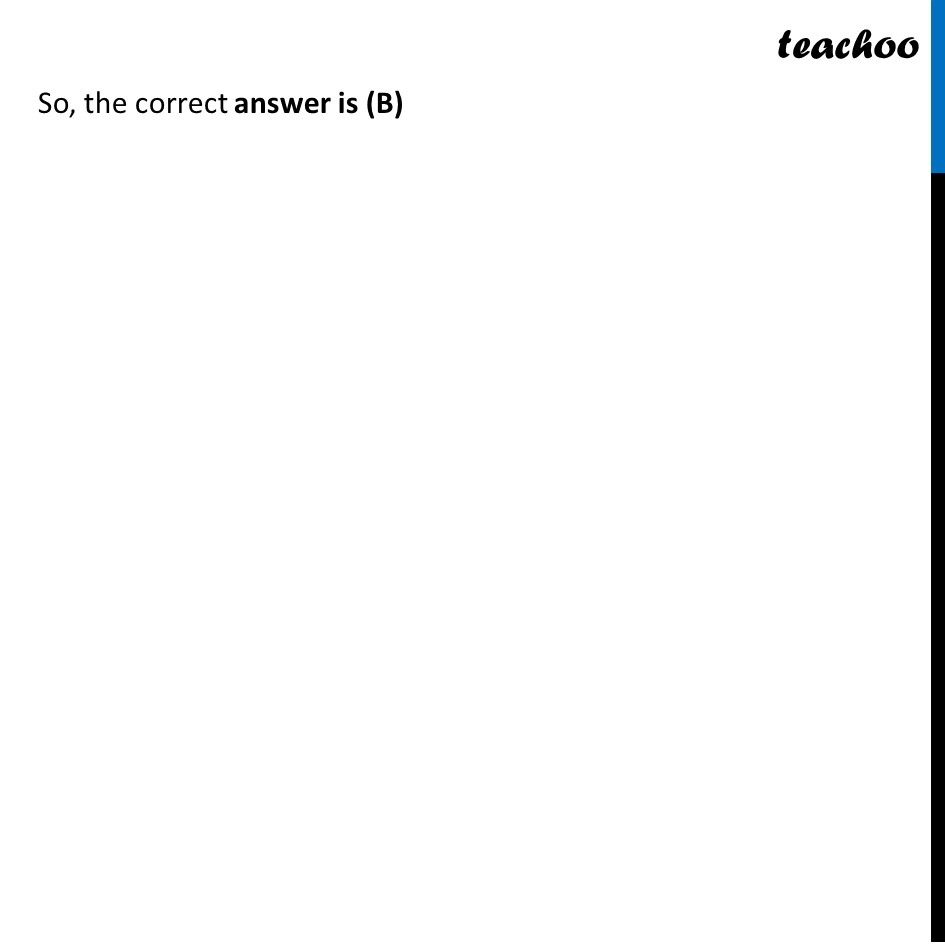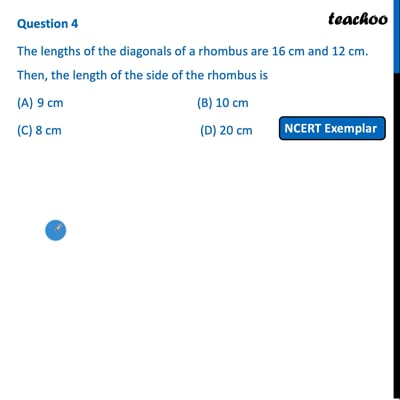NCERT Exemplar - MCQ

Chapter 6 Class 10 Triangles
Serial order wise

## (D) 20 cm

This question is inspired from  Ex 6.5, 7 - Chapter 6 Class 10 - TrianglesThis video is only available for Teachoo black users

Learn in your speed, with individual attention - Teachoo Maths 1-on-1 Class

### Transcript

Question 4 The lengths of the diagonals of a rhombus are 16 cm and 12 cm. Then, the length of the side of the rhombus is 9 cm (B) 10 cm (C) 8 cm (D) 20 cm In a Rhombus Sum of squares of sides = Sum of its diagonals (For proof, check Ex 6.5, 7 – Chapter 6 Class 10 Triangles) Thus, AB2 + BC2 + CD2 + AD2 = AC2 + BD2 Since all sides of Rhombus are equal, our equation becomes AB2 + AB2 + AB2 + AB2 = AC2 + BD2 4AB2 = AC2 + BD2 Putting length of diagonals as 16 and 12 cm 4AB2 = 162 + 122 4AB2 = 256 + 144 4AB2 = 400 AB2 = 400/4 AB2 = 100 AB2 = 102 AB = 10 cm So, the correct answer is (B) So, the correct answer is (B)